# Single-Image Depth Perception Net Trained onNYU Depth V2 Data

Estimate the depth map of an image

Released in 2016, this neural net was trained to predict the relative depth map from a single image using a novel technique based on sparse ordinal annotations. Each training example only needs to be annotated with a pair of points and its relative distance to the camera. After training, the net is able to reconstruct the full depth map. Its architecture is based on the "hourglass" design.

Number of layers: 501 | Parameter count: 5,385,185 | Trained size: 23 MB |

## Training Set Information

• NYU Depth V2, consisting of 1,449 RGBD indoor images with dense per-pixel semantic and structural labeling.

## Performance

• This model achieves 28.3% WKDR (Weighted Kinect Disagreement Rate) error on the NYU Depth V2 dataset and 31.31% WHDR (Weighted Human Disagreement Rate) error on the Depth in the Wild dataset. All weights are set to 1 for both measures.

## Examples

### Resource retrieval

Get the pre-trained net:

 In:=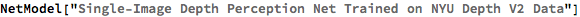Out=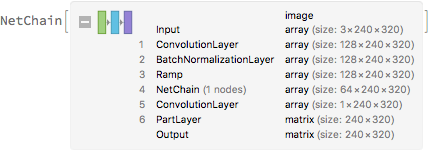### Basic usage

Obtain the depth map of an image:

 In:=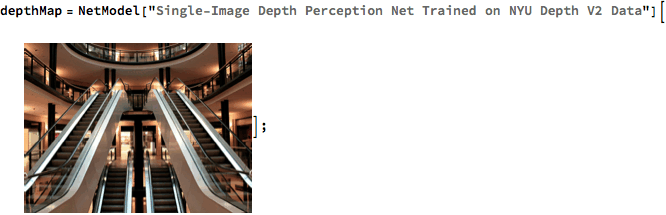Show the depth map:

 In:=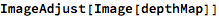Out=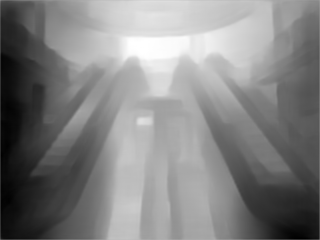### Visualize a 3D model

Get an image:

 In:=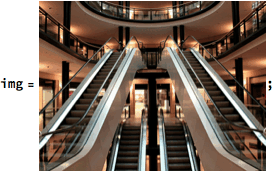Obtain the depth map:

 In:=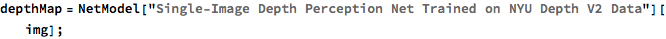Visualize a 3D model using the depth map:

 In:=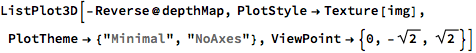Out=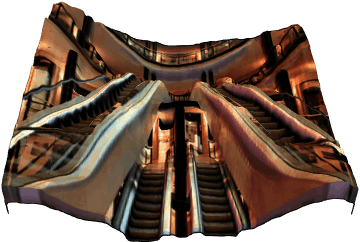The recommended way to deal with image sizes and aspect ratios is to resample the depth map after the net evaluation. Get an image:

 In:=Obtain the dimensions of the image:

 In:=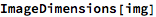Out=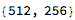Obtain the depth map

 In:=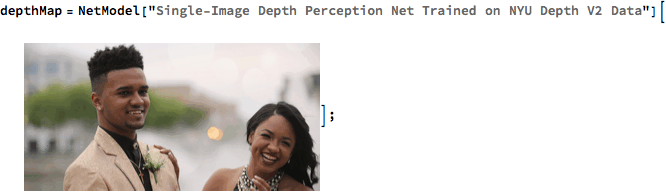Resample the depth map and visualize it:

 In:=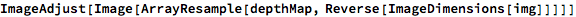Out=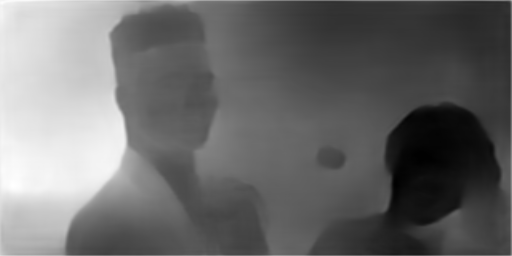### Net information

Inspect the number of parameters of all arrays in the net:

 In:=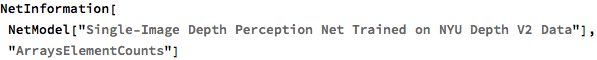Out=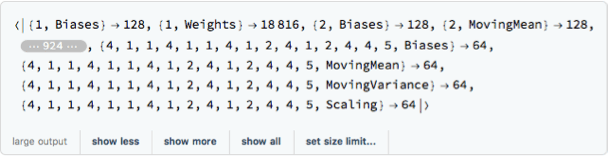Obtain the total number of parameters:

 In:=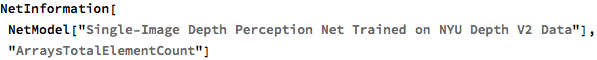Out=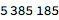Obtain the layer type counts:

 In:=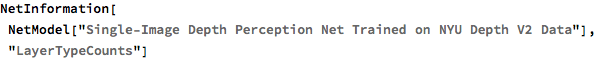Out=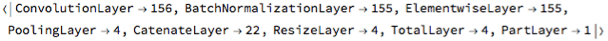Display the summary graphic:

 In:=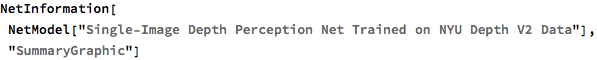Out=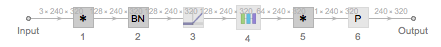### Export to MXNet

Export the net into a format that can be opened in MXNet:

 In:=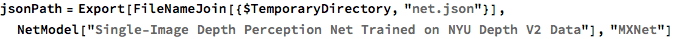Out=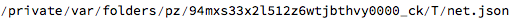Export also creates a net.params file containing parameters:

 In:=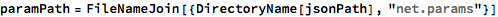Out=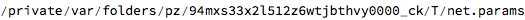Get the size of the parameter file:

 In:=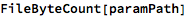Out=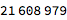The size is similar to the byte count of the resource object:

 In:=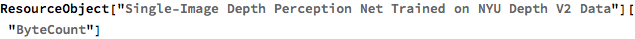Out=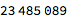## Requirements

Wolfram Language 11.2 (September 2017) or above